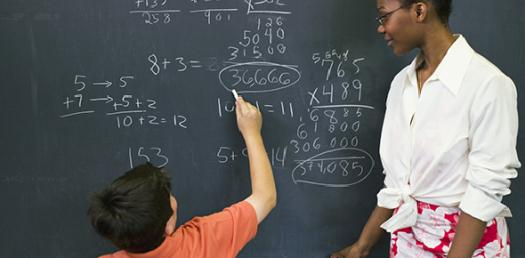# Math Trivia For 5th Grade

15 Questions | Attempts: 530
ShareSettingsHere are some amazing quiz questions on 5th grade math

• 1.
If you burn 100 calories by climbing 100 stairs, how many calories do you burn climbing 50 stairs?
• A.

150

• B.

10

• C.

50

• D.

25

• 2.
What is 36% of 40?
• A.

14.4

• B.

144

• C.

25.6

• D.

1440

• 3.
What do we call a triangle with exactly two equal sides?
• A.

Equilateral Triangle

• B.

Scalene Triangle

• C.

Isosceles Triangle

• D.

Equilateral Triangle

• 4.
What do we call a triangle that has all three sides of equal length?
• A.

Equilateral Triangle

• B.

Isosceles Triangle

• C.

Scalene Triangle

• D.

Right Triangle

• 5.
How many inches are in 4 feet?
• A.

12

• B.

16

• C.

32

• D.

48

• 6.
What do we call a triangle with no equal sides?
• A.

Right Triangle

• B.

Isosceles Triangle

• C.

Equilateral Triangle

• D.

Scalene Triangle

• 7.
Which of the following numbers is a factor of 14?
• A.

3

• B.

7

• C.

10

• D.

5

• 8.
What do the numbers 1, 16 and 49 all have in common?
• A.

They Are All Square Numbers

• B.

They Are All Divisible By 7

• C.

They Are All Prime Numbers

• D.

They Are All Odd Numbers

• 9.
What is the value of 3 with an exponent of 4?
• A.

75

• B.

12

• C.

7

• D.

81

• 10.
Which of these numbers is largest?
• A.

0.325

• B.

0.3225

• C.

0.316

• D.

0.3249

• 11.
A bullfrog is 20.3 cm long. The American toad is 11 cm long. How much longer is the bullfrog?
• A.

1.92 Cm

• B.

19.2 Cm

• C.

9.3 Cm

• D.

.93 Cm

• 12.
What is 5813 - 3925?
• A.

1788

• B.

1898

• C.

1878

• D.

1888

• 13.
What is the least common multiple of 8 and 12?
• A.

48

• B.

96

• C.

72

• D.

24

• 14.
What is 387,796 rounded to the nearest thousand?
• A.

390,000

• B.

387,000

• C.

380,000

• D.

388,000

• 15.
What is the least common multiple of 10 and 25?
• A.

100

• B.

25

• C.

10

• D.

50

## Related TopicsBack to top
×

Wait!
Here's an interesting quiz for you.